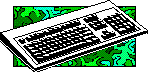# 3D Fractal Landscapes

The first time I heard the word "fractal" was a little over thirteen years ago, when I was still a mainframe programmer. A coworker and I were talking about our spiffy new IBM PC’s, and he asked me if I had any fractal software. "No, what are fractals?"

He explained that you made a fractal by applying some geometric operation to a simple figure, and then repeatedly applying the same operation to the result. While this explanation doesn’t even begin to touch on the sort of mathematical properties of fractals that interest mathematicians, it remains a good summary of how most fractals are generated.

It certainly describes 3D fractal landscape generation.

To generate a landscape, simply assign random heights to the three vertices of an equilateral triangle, then ‘bend’ each of the edges by raising or lowering the midpoint by some random amount. If you draw lines between each of the three midpoints, you have carved the initial triangle into four triangles. If you apply this bending and dividing operation to each new triangle as you create it, before long you will end up with something that looks surprisingly like a real landscape. (Figures 1, 2, and 3.)

## The devil’s in the details

Now, of course fractal landscape generation’s not quite as simple as "bend, divide, repeat" - you have to make sure that you only bend a given line once, and of course you do generally want to display the landscape - but those are the details.

### No floating triangles

The first and most important detail is that you have to keep track of what you’re doing. If you straightforwardly write the FractureTriangle() routine to bend each edge, you will end up with something like figure 4. Your triangles won’t form a smooth mesh; each group of four triangles will ‘float’ with each vertex at a different height than its neighbors’.

A look at figure 5 may help make it clear what’s going on. Vertex I is the midpoint of the line DF, which is shared by triangles CDF and DEF. If both triangles try to set the height of I, triangles 1, 2, and 3 will be given a different height for I than triangles 4, 5, and 6!

Clearly, we need to maintain a database of vertices, so that we can set vertex I when we fracture triangle CDF - and use the same value for vertex I when we fracture triangle DEF.

We might try to say that triangle DEF is an "inside" triangle, and that therefore we will fracture it last and use the "outer" triangles’ values for vertices G, H, and I - but look at triangles GEH and LMN. Lines GE and EH are shared with "outside" triangles, so we should use the outside triangles’ values for vertices L and M, but line GH is "all inside", so we need to bend it. We could undoubtedly elaborate the scheme of inside and outside triangles to handle these "outside inside subtriangles" properly, but we’d end up with code that was hard to read and liable to break whenever it was changed.

It’s far simpler to define a special value that only uninitialized vertices will have and make FractureTriangle() look to see if any given midpoint has already been set. If it has, it uses that height; if it hasn’t, it gives it a new height. It may be a bit slower to calculate and lookup the midpoints of inside triangles than to simply pass in the right arguments, but the code is smaller and clearer - and displaying a landscape certainly takes much longer than generating it.

### Triangular array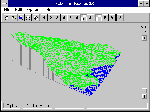Figure 1: An outlined fractal landscape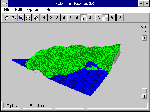Figure 2: A filled fractal landscape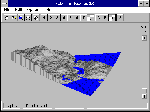Figure 3: A rendered fractal landscape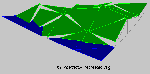Figure 4: When edges don't meet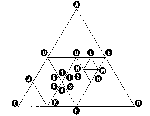Figure 5: How triangles 'fight' over edges

Now, when we bend a line, we are only changing the midpoint’s z value, so in principle we could somehow use the [xy] coordinate pair as an index into a table of z values. However, this would turn out to be a very sparse array, and the code will run a lot faster if we can use a normal, contiguous array than if we have to keep walking a sparse array’s lists. This is why Listing 1 defines a system of 2D logical addresses - the TVertex datatype - which ‘name’ the actual 3D coordinates - the TTriple datatype.

Listing 1: Vertices and Triples

Perhaps the simplest naming scheme is to simply number each vertex along the outermost triangle’s three edges, as in figure 6, and use all three coordinates to refer to each edge. While we really only need two coordinates and the third is redundant, I find it a bit clearer to be able to refer to the three outermost vertices as [1, 0, 0], [0, 1, 0], and [0, 0, 1] rather than as [1, 0], [0, 1], and [0, 0]. That’s why a TVertex is defined as a coordinate triple, even though the third coordinate is not really necessary and does slow things down a bit.

When it comes to the database of vertices, though, the third coordinate is ignored. As you can see from figure 6, each vertex has the same coordinates if we make the equilateral triangle into an isosceles triangle. This lets us use the AB and BC coordinates much as if they were the normal row and column coordinates of a square array.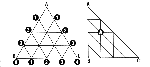Figure 6: Each vertex has the same coordinates in the equilateral triangle and the right triangle

However, if we did store our triangular array in a square array, we would be wasting nearly half the space. This wouldn’t be such a big deal, except that in sixteen bit environments we run into the 64K barrier. Each TTriple consists of three 16-bit fixed-point numbers, so a square array for an eight ply database (figure 7) would take (28-1+1)2 vertices, or 99,846 bytes. If we store only the cells on and below the diagonal, we can pare this to 50,310 bytes. This lets us use simple array indexing instead of arrays of arrays or huge pointers. Similarly, at least in this demo program, the database will fit in the data segment, which does makes for faster access than if we had to use heap blocks and pointers.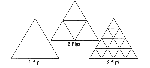Figure 7: The number of 'plys' corresponds to the number of recursive subdivisions of the original triangle.

Since eight plys is hardly too fine a resolution for, say, a 1280 by 1024 screen, the demo program for this article, Fractal Landscapes 3.0, [a Delphi port of a Windows port of a DOS program, which was originally written as a ‘cute hack’ in Turbo Pascal 4.0] uses the triangular database code in Listing 2. The basic idea is that each row of vertices follows right after the previous row. Since there is only one vertex on the first row, the second row starts in DB’s second ‘cell’. Since the second row has two vertices, the third row starts in DB’s fourth cell, and so on.

Listing 2: Triangular database code

### Bending

Another subtlety that I, at least, didn’t discover until I actually wrote the code is that you shouldn’t apply the same amount of randomness when you bend the larger scale lines as when you bend the smaller scale lines. If you do, you either end up with a bumpy plane or a spiky landscape. You need to apply more randomness to the large outer triangles, which produce the overall shape of the landscape, and to apply less randomness to the smaller inner triangles, which basically control the smoothness of your landscape.

What I ended up using is a function that generates something vaguely like a normal distribution

```function Rand(Envelope: integer): integer;
{ Pseudonormal (sawtooth) distribution,
in range ±Envelope }
begin
Rand := integer(Random(Envelope)) +
integer(Random(Envelope)) -
Envelope;
end;```
where the Envelope for each ply is half that of the next larger ply. This certainly produces plausible looking landscapes, but real landscapes aren’t always as smooth as FL3’s. Real landscapes do have the occasional sharp edge - cliffs, mesas, canyons, and so on - while FL3 never really produces anything more abrupt than a steep slope.

One approach you may want to experiment with is to replace Rand’s pseudonormal distribution within a constricting envelope with an exponential function. On smaller scales, the function would be more likely to produce a number close to 0 than on larger scales, but it might throw out a large number on any scale.

### Draw then display

In the first incarnation of this program, the same recursive routine that built the landscape was responsible for drawing it. If the Plys argument was greater than 1, it broke its input triangle into four new triangles, and then decremented Plys and applied itself to each new triangle. When the Plys argument was equal to 1, it called a routine that drew the triangle.

This was certainly simple enough, but it meant that changing from a ‘wire mesh’ rendering to a filled-triangle rendering required generating a whole new landscape. Similarly, using this simple design in a Windows version would mean that changing the window size also generates a whole new landscape. Clearly, a better approach is to generate the landscape then draw it. This requires two parallel recursions from the outermost triangle to the innermost ones (which are the only ones which are actually drawn), but the second recursion doesn’t cost much compared to actually drawing the rectangles, so the price of flexibility is rather low.

### And now, the code

After all that prolog, the actual generation code in Listing 3 may seem refreshingly simple.

FractureTriangle() takes a triangle and the number of Plys remaining. If Plys is greater than 1, FractureTriangle() calls FractureLine() to create (or retrieve) a midpoint value, then calls itself on each of the four triangles that these midpoints define. FractureLine() calls Midpoint() to calculate the vertex between its two input vertices, and then checks to see if it has been set yet. If the midpoint is still uninitialized, FractureLine() bends the line between the endpoints by raising or lowering its midpoint.

## Displaying the landscape

Listing 3: Fracturing lines and triangles

Once the landscape has been generated, FL3 uses the code in Listing 4 to display it in the current window, in the current display mode. If the user changes the window size or the display mode, FL3 redraws the landscape.

There are three display modes: Outline, Filled, and ‘Rendered’. All three display the landscape as a collection of triangles by doing a single-point perspective transform of the triangle’s three TTriple-s to screen TPixel-s, then doing either a PolyLine or Polygon draw. The only difference between the Outline mode and Filled and Rendered modes is that where Outline mode draws a simple ‘wire mesh’ picture of the landscape with no hidden line removal, Filled and Rendered mode rely on drawing order and polygon filling to do a brute force hidden line removal. Rendered mode in turn differs from Filled mode in basing each triangle’s color on the angle it presents to a ray from the ‘sun’.

Listing 4: Display code

Draw3Vertices() implements a simplistic concept of "sea level" to lend a bit of verisimilitude to the display. Any triangle that’s completely above sea level is drawn normally, while any triangle that’s completely below sea level, is drawn in blue, at sea level. If the triangle crosses sea level, FL3 interpolates the crossing points, and draws part of the triangle above water and part below. While this is plausible enough for "coastlines", it’s a bit less plausible for lakes: FL3 doesn’t detect the lowest lip of a basin, but colors as water only the part(s) of a basin that are under sea level.

Once all the triangles are drawn, FL3 draws vertical lines along the two front edges from sea level to any vertices which are above sea level. This is particularly effective in the two filled modes, where the filled vertical quadrilaterals obscure the undersides of the landscape surface.

### The Project() routine

The Project() routine is the workhorse on which all drawing operations depend. It converts a 3D TTriple to a 2D TPixel using single point perspective and the current window dimensions.

In effect, it draws a line through the point and a vanishing point, and marks the intersection of that line and the screen. Most of the complexity in Listing 4 comes from the use of fixed-point math. (This is primarily a legacy of FL3’s origins in the days when float point math was very slow, but it does also serve to reduce the vertex database’s size to the point where it fits in the data segment.) If we strip away the fixed point math, we end up with

```function Project(const P: TTriple): TPixel;
{ 3D transform a point }
var
Ratio: double;
Pt, V: TFloatTriple;
begin
Pt := FloatTriple(P);
V  := FloatTriple(VanishingPoint);

Ratio    := Pt.Y / V.Y;
Result.X := Round( DisplayWidth *
((V.X - Pt.X) * Ratio + Pt.X));
Result.Y := DisplayHeight -
Round( DisplayHeight *
((V.Z - Pt.Z) * Ratio + Pt.Z));
end;```
That is, it calculates the ratio of the point’s depth to the vanishing point’s depth, and applies this to the difference between the point’s x and z coordinates and the vanishing point’s x and z coordinates. Since the TTriple coordinate system ranges from 0 to 1, we can simply multiply the projected coordinates by the window size to obtain the proper screen coordinates.

### Outline mode

Outline mode is the simplest of all the drawing modes. It simply outlines ‘land’ triangles in green and water triangles in blue. (Figure 1) About all that’s worth really noting here is how simple and clear Delphi makes DrawPixels(). The API version of DrawPixels would replace the single, simple statement "Canvas.PolyLine( [A, B, C, A] )" with a local array declaration and four assignments - not to mention all the bother over creating and destroying DC’s and pens.

### Filled mode

Outline mode is relatively fast and does a pretty good job of suggesting texture, but it has one big problem: you can see right through it. This means that the mesh on the backside of a hill, say, shows through to the front side. Sophisticated graphics applications have complicated algorithms for "hidden line removal", but FL3 is not sophisticated, and relies on brute force to remove hidden lines by drawing over them. (Figure 2)

That is, DrawTriangle() takes care to draw the rearmost triangles first, so that any triangles in front will be drawn later, obscuring the triangles in back. On the initial call to DrawTriangle(), the triangle is ‘point down’. Vertex A is closest to the front and the bottom of the window, and B and C are in back, towards the top of the window. (Figure 8) Thus,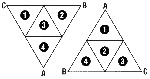Figure 8: Drawing order and triangle orientation

```DrawTriangle(Canvas, CA, BC, C, Plys, True);
DrawTriangle(Canvas, AB, B, BC, Plys, True);
DrawTriangle(Canvas, BC, CA, AB, Plys, False);
DrawTriangle(Canvas, A, AB, CA, Plys, True)```

draws the leftmost subtriangle first, then the rightmost. Both of these ‘outside’ subtriangles face the same way as triangle ABC, and the order of the parameters to the recursive call to DrawTriangle() ensures that the new A will also be in front, with the new B and C in back.

The third call draws the ‘inside’ subtriangle, as this is visually in front of the second, rightmost triangle. In every triangle, the inside subtriangle is upside down to its outer triangle, so on this initial call, the inside triangle is ‘point up’. The parameter ordering ensures that on point up calls, A is still the ‘point’, facing up, with B and C in front, towards the bottom of the screen. If you step through DrawTriangle()’s not PointDn set of recursive calls, you will see that point up triangles are drawn back to front, right to left.

The fourth call draws the last, frontmost subtriangle.

### Rendered mode

Rendered mode attempts to look more realistic than filled mode by coloring each triangle based on the angle between the triangle and a ray from the ‘sun’, so that triangles orthogonal to the rays from the sun are lighter than triangles that present a more oblique angle to the sun’s rays. (Figure 3) While it does seem to do a good job of showing, say, the curvature of a basin, overall it’s a bit ... gray. You may wish to experiment with a palette and LandColor() function that use a bit of false color.

## Wrapup

FL3 is a simple demo of fractal landscape generation techniques. You may wish to try to improve the random number distribution or the rendering code, or to modify the algorithm so as to generate entire fractal planets.

It may not be obvious that ‘plasma’ routines are basically just a rectangular version of a fractal landscape generation algorithm: They fracture rectangles instead of triangles, and use color to show the 3rd dimension instead of perspective.

The four printed listings include only the most important pieces of the program, which is too large to print in full in a magazine. The full Delphi source and executable are available on-line and via the code disk.

 Jon Shemitz has been a Pascal/Delphi consultant and independent developer for nearly thirteen years. He lives in Santa Cruz, California with his partner and their two children, and is contemplating buying a laptop so he can work in his greenhouse. He can be reached at jon@midnightbeach.com or via http://www.midnightbeach.com/jon. This article first appeared in Visual Developer magazine Copyright © 1996..1997 Jon Shemitz - jon@midnightbeach.com - January 13, 1996..June 23, 1997